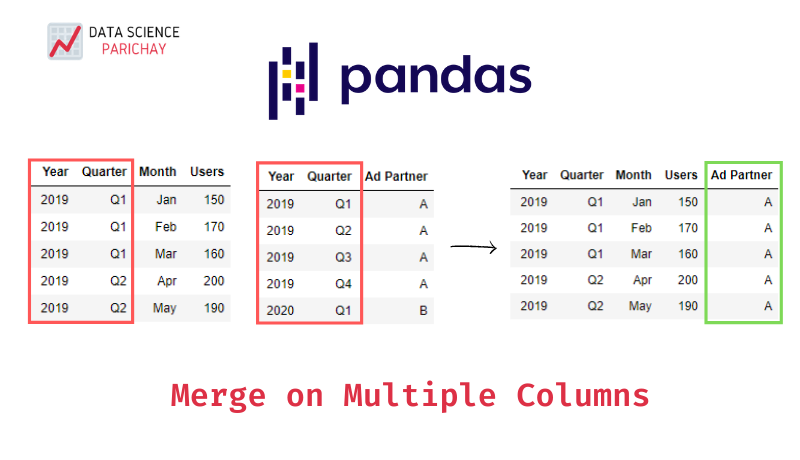# Pandas – Merge DataFrames on Multiple Columns

In this tutorial, we’ll look at how to merge pandas dataframes on multiple columns.

The pandas `merge()` function is used to do database-style joins on dataframes. To merge dataframes on multiple columns, pass the columns to merge on as a list to the `on` parameter of the `merge()` function. The following is the syntax:

``df_merged = pd.merge(df_left, df_right, on=['Col1', 'Col2', ...], how='inner')``

Note that, the list of columns passed must be present in both the dataframes. If the column names are different in the two dataframes, use the `left_on` and `right_on` parameters to pass your column lists to merge on.

Let’s look at an example of using the `merge()` function to join dataframes on multiple columns. First, let’s create two dataframes that we’ll be joining together.

``````import pandas as pd

# monthly users
df_users = pd.DataFrame({
'Year': [2019,2019,2019,2019,2019,2019,2019,2019,2019,2019,2019,2019,2020,2020,2020],
'Quarter': ['Q1','Q1','Q1','Q2','Q2','Q2','Q3','Q3','Q3','Q4','Q4','Q4','Q1','Q1','Q1'],
'Month': ['Jan','Feb','Mar','Apr','May','Jun','Jul','Aug','Sep','Oct','Nov','Dec','Jan','Feb','Mar'],
'Users': [150,170,160,200,190,196,210,225,260,210,212,219,630,598,321]
})

'Year': [2019,2019,2019,2019,2020],
'Quarter': ['Q1','Q2','Q3','Q4','Q1']
})``````

The dataframe `df_users` shows the monthly user count of an online store whereas the table `df_ad_partners` shows which ad partner was handling the store’s advertising.

If we want to include the advertising partner info alongside the users dataframe, we’ll have to merge the dataframes using a left join on columns “Year” and “Quarter” since the advertising partner information is unique at the “Year” and “Quarter” level.

``df_merged = pd.merge(df_users, df_ad_partners, on=['Year', 'Quarter'], how='left')``

This is the dataframe we get on merging –

You can see the Ad Partner info alongside the users count. An interesting observation post the merge is that there has been an increase in users since the switch from A to B as the advertising partner.

📚 Data Science Programs By Skill Level

Introductory

Intermediate ⭐⭐⭐

🔎 Find Data Science Programs 👨‍💻 111,889 already enrolled

Disclaimer: Data Science Parichay is reader supported. When you purchase a course through a link on this site, we may earn a small commission at no additional cost to you. Earned commissions help support this website and its team of writers.

In the above example, we saw how to merge two pandas dataframes on multiple columns. The columns to merge on had the same names across both the dataframes. It can happen that sometimes the merge columns across dataframes do not share the same names. In that case, you can use the `left_on` and `right_on` parameters to pass the list of columns to merge on from the left and right dataframe respectively.

For a complete list of pandas `merge()` function parameters, refer to its documentation.

With this, we come to the end of this tutorial. The code examples and results presented in this tutorial have been implemented in a Jupyter Notebook with a python (version 3.8.3) kernel having pandas version 1.0.5

•